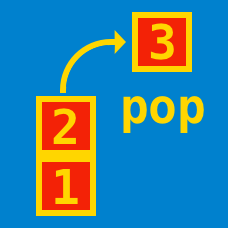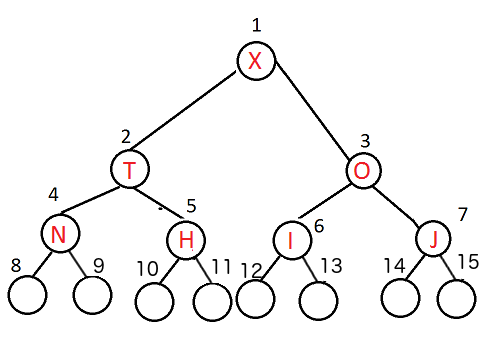Computer Science

# Heap/Priority Queue

A binary min-heap is made by including each integer $[1, 2000]$ exactly once. The depth of a node in the heap is the length of the path from the root of the heap to that node. What is the maximum depth at which the number $11$ can appear?

Details and Assumptions

-The root is at depth $0$

Consider a complete binary heap where the element with the highest priority is kept at the root of the heap.

Suppose we define a method insert(value) that inserts the new element with the highest priority into the queue.

What is the run time for insert(8)?

Consider the following tree representation of a heap. It is graphical representation of an array based max-heap and the numbers represent indices of the array, while the alphabets are the actual keys of the heap.Suppose that we want to insert the letter 'L' into this new heap. Which of the following indices could it possibly have without violating the heap condition?

×